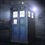# Prove/Disprove Combinatorics 1

Prove/Disprove:

If $3p$ distinct elements are divided in three groups each containing $p$ elements.then the number of divisions is $\dfrac{(3p)!}{(p!)^3}$.5 years, 5 months ago

This discussion board is a place to discuss our Daily Challenges and the math and science related to those challenges. Explanations are more than just a solution — they should explain the steps and thinking strategies that you used to obtain the solution. Comments should further the discussion of math and science.

When posting on Brilliant:

• Use the emojis to react to an explanation, whether you're congratulating a job well done , or just really confused .
• Ask specific questions about the challenge or the steps in somebody's explanation. Well-posed questions can add a lot to the discussion, but posting "I don't understand!" doesn't help anyone.
• Try to contribute something new to the discussion, whether it is an extension, generalization or other idea related to the challenge.

MarkdownAppears as
*italics* or _italics_ italics
**bold** or __bold__ bold
- bulleted- list
• bulleted
• list
1. numbered2. list
1. numbered
2. list
Note: you must add a full line of space before and after lists for them to show up correctly
paragraph 1paragraph 2

paragraph 1

paragraph 2

[example link](https://brilliant.org)example link
> This is a quote
This is a quote
    # I indented these lines
# 4 spaces, and now they show
# up as a code block.

print "hello world"
# I indented these lines
# 4 spaces, and now they show
# up as a code block.

print "hello world"
MathAppears as
Remember to wrap math in $$ ... $$ or $ ... $ to ensure proper formatting.
2 \times 3 $2 \times 3$
2^{34} $2^{34}$
a_{i-1} $a_{i-1}$
\frac{2}{3} $\frac{2}{3}$
\sqrt{2} $\sqrt{2}$
\sum_{i=1}^3 $\sum_{i=1}^3$
\sin \theta $\sin \theta$
\boxed{123} $\boxed{123}$

Sort by:

- 5 years, 5 months ago

Let there be n = a+b+c distinct things.Find the number of ways of dividing these n things in groups containing a,b,c things.

- 5 years, 5 months ago

(a+b+c)!/3!a!b!c!

- 5 years, 5 months ago

Correct, prove it.

- 5 years, 5 months ago

$\dbinom{3p}{p} \dbinom{2p}{p} \dbinom{p}{p} = \dfrac{(3p)!}{(p!)^{3}}$

Out of 3p things, we can first choose p things, then outta remaining 2p things, we can choose p things and out of remaining p things we can choose p things in 1 one way only.

- 5 years, 5 months ago

@Nihar Mahajan and@Harsh Shrivastava try to find the mistake using the example.

Let p =1(elements be 1,2,3) then n.o of different groups is 1 but according to your generalization its coming 3!.

- 5 years, 5 months ago

Yup , I'll rectify my solution later, a bit buzy now,

I forgot that we also have to divide by 3! since there are repetitions.

- 5 years, 5 months ago

"Divide by 2"?

- 5 years, 5 months ago

Errr fixed typo.

- 5 years, 5 months ago

Try the second one I have posted!

- 5 years, 5 months ago

It will be divided by 3! .There was a typo in my comment

- 5 years, 5 months ago

And I think that is because the groups are identical,so we don't need to permute them.

- 5 years, 5 months ago

Could you elaborate a bit more?What repetitions are you talking about?

- 5 years, 5 months ago

I am sorry to say but that is incorrect,just think it up for some time.It is a very common mistake.

- 5 years, 5 months ago

Without any restriction , the $3p$ elements can be arranged in $(3p)!$ . Now we need to compute the total repetition factor. In each group , the $p$ elements can be permuted in $p!$ ways , so by multiplication principle in three groups, total repetition factor $=(p!)^3$ . Hence , number of divisions $=\frac{(3p)!}{(p!)^3}$ .

(I may be wrong)

- 5 years, 5 months ago

No,sorry,just go through the discussion once.

- 5 years, 5 months ago